Jump to content# Getting a point of an entity inside a block as WCS coordinates

## Recommended PostsI have a circle inside a block and this block is inserted in a drawing. I want to get the coordinates of the center of that circle in WCS. Obviously, the center of the circle is not the insertion point.

I found 'nentselp' to transform with a trans. matrix but requires me to give a point which I don't have (user input is out of the question) so I doesn't work for me. All other data of the block is however known.

I managed to get the center of the circle by using the following line

`(setq ptz (cdr (assoc 10 (entget (cadr (getblkitems ent))))))`

The 'getblkitems' returns all entity names in a list (somebody kindly posted in a forum) and I know the second entity (hence the cadr above) is the circle.

But now the question, I don't have the trans. matrix to get the point in WCS. Any ideas how this could be returned transformed or any ideas of a different approach?

Thank you.

#### Share this post

##### Share on other sitesHere is an example:

```(defun blockreferencecirclecenter ( ref / cen ent enx )
(setq ent (tblobjname "block" (cdr (assoc 2 (entget ref)))))
(while
(and
(null cen)
(setq ent (entnext ent))
(setq enx (entget  ent))
)
(if (= "CIRCLE" (cdr (assoc 0 enx)))
(setq cen (trans (cdr (assoc 10 enx)) (cdr (assoc 210 enx)) 0))
)
)
(if cen (apply '(lambda ( mat vec ) (mapcar '+ (mxv mat cen) vec)) (refgeom ref)))
)

;; RefGeom (gile)
;; Returns a list whose first item is a 3x3 transformation matrix and
;; second item the object insertion point in its parent (xref, block or space)

(defun refgeom ( ent / ang enx mat ocs )
(setq enx (entget ent)
ang (cdr (assoc 050 enx))
ocs (cdr (assoc 210 enx))
)
(list
(setq mat
(mxm
(mapcar '(lambda ( v ) (trans v 0 ocs t))
'(
(1.0 0.0 0.0)
(0.0 1.0 0.0)
(0.0 0.0 1.0)
)
)
(mxm
(list
(list (cos ang) (- (sin ang)) 0.0)
(list (sin ang) (cos ang)     0.0)
'(0.0 0.0 1.0)
)
(list
(list (cdr (assoc 41 enx)) 0.0 0.0)
(list 0.0 (cdr (assoc 42 enx)) 0.0)
(list 0.0 0.0 (cdr (assoc 43 enx)))
)
)
)
)
(mapcar '- (trans (cdr (assoc 10 enx)) ocs 0)
(mxv mat (cdr (assoc 10 (tblsearch "block" (cdr (assoc 2 enx))))))
)
)
)

;; Matrix Transpose  -  Doug Wilson
;; Args: m - nxn matrix

(defun trp ( m )
(apply 'mapcar (cons 'list m))
)

;; Matrix x Matrix  -  Vladimir Nesterovsky
;; Args: m,n - nxn matrices

(defun mxm ( m n )
((lambda ( a ) (mapcar '(lambda ( r ) (mxv a r)) m)) (trp n))
)

;; Matrix x Vector  -  Vladimir Nesterovsky
;; Args: m - nxn matrix, v - vector in R^n

(defun mxv ( m v )
(mapcar '(lambda ( r ) (apply '+ (mapcar '* r v))) m)
)```

...And a program to test it:

```(defun c:test ( / cen ent )
(if (setq ent (car (entsel)))
(if (= "INSERT" (cdr (assoc 0 (entget ent))))
(if (setq cen (blockreferencecirclecenter ent))
(entmake (list '(0 . "POINT") (cons 10 cen) (cons 210 (trans '(0 0 1) 1 0 t))))
(princ "\nNo circle found in block definition.")
)
(princ "\nObject is not a block.")
)
(princ "\nNo object selected.")
)
(princ)
)```

#### Share this post

##### Share on other sites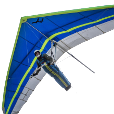... But now the question, I don't have the trans. matrix to get the point in WCS. Any ideas how this could be returned transformed or any ideas of a different approach?

Thank you.

If you know the coordinates of the point in the coordinate system of the block (pOCS in the example below), or can get them, you can multiply the point's homogeneous coordinates by the matrix. My matrix multiply function is not as elegant as some others but it works.

```(defun c:tt (/)
(setq pOCS '(1.11 2.22 3.33))  ; coordinates in Object Cor. Sys
(setq M (cadr (cdr (nentsel)) )) ; block 4 x 3 matrix

(setq pWCS (transform pOCS M)) ; coordinates in WCS
(princ pwcs)
(princ)
)

(defun transform (a HTM / transpt)	;Coordinate transformation via matrix multiplication
; a = point (3 coordinates)
; HTM = homogeneous transformation matrix (3 x 4)
(setq aH (append a '(1.0)))		; aH = homogeneous point in local coordinates (1 x 4)
(setq	transpt
(list
(+				; transpt = list of x, y, z coordinates
(* (nth 0 aH) (nth 0 (nth 0 HTM)))
(* (nth 1 aH) (nth 0 (nth 1 HTM)))
(* (nth 2 aH) (nth 0 (nth 2 HTM)))
(* (nth 3 aH) (nth 0 (nth 3 HTM)))
)
(+
(* (nth 0 aH) (nth 1 (nth 0 HTM)))
(* (nth 1 aH) (nth 1 (nth 1 HTM)))
(* (nth 2 aH) (nth 1 (nth 2 HTM)))
(* (nth 3 aH) (nth 1 (nth 3 HTM)))
)
(+
(* (nth 0 aH) (nth 2 (nth 0 HTM)))
(* (nth 1 aH) (nth 2 (nth 1 HTM)))
(* (nth 2 aH) (nth 2 (nth 2 HTM)))
(* (nth 3 aH) (nth 2 (nth 3 HTM)))
)
)
)
(setq transpt (list (nth 0 transpt) (nth 1 transpt) (nth 2 transpt)))
)					;end transform ```

There's probably a way to use the trans function to do this.

LRM

#### Share this post

##### Share on other sites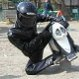@ Lee. Mind. Blown.Seems long tho. An alternative inspired by another piece of art by the great Lee Mac.

```(defun c:test ( / ent sel lst)
(if (and (cdddr (setq sel (nentselp)))
(= "CIRCLE" (cdr (assoc 0 (setq lst (entget (car sel))))))
(setq ent (entmakex (list '(0 . "POINT") (cons 10 (trans (cdr (assoc 10 lst)) (cdr (assoc 210 lst)) 0)) (cons 210 (trans '(0 0 1) 1 0 t)))))
)
(progn
(vla-transformby (vlax-ename->vla-object ent) (vlax-tmatrix (caddr sel)))
(vla-regen (vla-get-activedocument (vlax-get-acad-object)) acallviewports)
)
)
(princ)
)```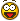#### Share this post

##### Share on other sites@ Lee. Mind. Blown.Cheers Jef!

Seems long tho. An alternative inspired by another piece of art by the great Lee Mac.

Thanks for sharing, but as stated by the OP:

I found 'nentselp' to transform with a trans. matrix but requires me to give a point which I don't have (user input is out of the question) so I doesn't work for me. All other data of the block is however known.

...else I would also have suggested nentsel(p)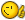#### Share this post

##### Share on other sitesAh! And while in your code (c:test) you could replace the (car(entsel by any ename, I cannot feed an insert's ename in any way to (nentselp) in my alternative to remove the user input, right? I see.

I was about to add some little wheels on my avatar, I think I will postpone and keep my rig as is for a little while longer!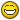Cheers

#### Share this post

##### Share on other sitesJust being curious:

Is it possible to obtain the block reference's matrix, without selecting it (with nentselp) , but using the ename/vla-object instead ?

I.e. writing a function that translates a provided point, which relies inside a block definition, and providing the block reference aswell (ofcourse) :

`(foo BlockReference PtInsideBlkDef) -> PtInWCS`

or even translate a whole pointlist (which might seem more efficient) :

`(foo BlockReference PtListInsideBlkDef) -> PtListInWCS`

I've had an old idea about obtaining the endpoints of a nested line/polyline's segment - in order to align two block references by picking nested lines/pline segments.

But I got stuck at this transformby task.

Ofcourse the concept of my idea might use (nentselp) picks , but my question is the 2nd row of my post.

Man you could even translate thru nested block references that way:

`(foo BlockReferenceA (foo BlockReferenceB (foo BlockReferenceC PtListInsideBlkDef)))`

If the answer to my question is "yes".#### Share this post

##### Share on other sitesAh! And while in your code (c:test) you could replace the (car(entsel by any ename, I cannot feed an insert's ename in any way to (nentselp) in my alternative to remove the user input, right? I see.

Exactly.I was about to add some little wheels on my avatar, I think I will postpone and keep my rig as is for a little while longer!

Unicycle?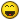Just being curious:

Is it possible to obtain the block reference's matrix, without selecting it (with nentselp) , but using the ename/vla-object instead ?

Yes - see this post.

Man you could even translate thru nested block references that way:

`(foo BlockReferenceA (foo BlockReferenceB (foo BlockReferenceC PtListInsideBlkDef)))`

If the answer to my question is "yes".

Indeed you can.

#### Share this post

##### Share on other sitesThanks Lee! - I guess I'll start investigating these codes more carefully.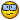#### Share this post

##### Share on other sitesOnce again, thanks Lee.

#### Share this post

##### Share on other sitesYou're welcome!

#### Share this post

##### Share on other sites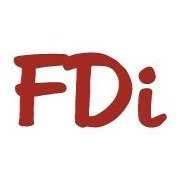Could be a lot of gottchas here

What if:

• The INSERT is not in WCS
• The INSERT is not equally scaled
• The INSERT is rotated
• The BLOCK INSBASE is not 0,0,0
• The CIRCLE is not WCS

A POINT entity would be the easiest, CIRCLE center fairly, The top point on an extruded CIRCLE at a given angle could pose some real problems

-David

#### Share this post

##### Share on other sitesCould be a lot of gottchas here

What if:

• The INSERT is not in WCS
• The INSERT is not equally scaled
• The INSERT is rotated
• The BLOCK INSBASE is not 0,0,0
• The CIRCLE is not WCS

A POINT entity would be the easiest, CIRCLE center fairly, The top point on an extruded CIRCLE at a given angle could pose some real problems

My example accounts for all of these.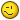#### Share this post

##### Share on other sitesOh wow !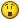## Join the conversation

You can post now and register later. If you have an account, sign in now to post with your account.
Note: Your post will require moderator approval before it will be visible.Reply to this topic...

×   Pasted as rich text.   Paste as plain text instead

Only 75 emoji are allowed.

×   Your link has been automatically embedded.   Display as a link instead

×   Your previous content has been restored.   Clear editor

×   You cannot paste images directly. Upload or insert images from URL.

×

×
×
• Create New...